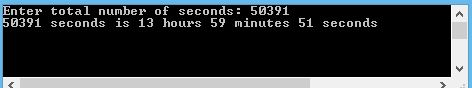SHARE
HELP

To start with, Sr2Jr’s first step is to reduce the expenses related to education. To achieve this goal Sr2Jr organized the textbook’s question and answers. Sr2Jr is community based and need your support to fill the question and answers. The question and answers posted will be available free of cost to all.Authors:
Walter Savitch ,kenrick Mock
Chapter:
C++ Basics
Exercise:
Programming Projects
Question:11 | ISBN:9780132846813 | Edition: 5

###Question

Write a program that inputs an integer that represents a length of time in seconds. The program should then output the number of hours, minutes, and seconds that corresponds to that number of seconds. For example, if the user inputs 50391 total seconds then the program should output 13 hours, 59 minutes, and 51 seconds.

###Answer

``````#include <iostream>
using namespace std;
int main( )
{
const unsigned int SECONDS= 60;

const unsigned int MINUTES= 60;
const unsigned int HOUR= 24;
const unsigned int DAY= 7;
unsigned long totalSeconds;
unsigned long weeks, dd, hr, minutes, seconds;
cout << "Enter total number of seconds: ";
cin >> totalSeconds;

//Calcualte the number of hours, minutes,
//and seconds
seconds = totalSeconds;
minutes = seconds / SECONDS;
seconds %= SECONDS;
hr = minutes / MINUTES;
minutes %= MINUTES;
dd = hr / HOUR;
hr %= HOUR;
weeks = dd / DAY;
dd %= DAY;
//Display output
cout << totalSeconds << " seconds is ";
if (weeks > 0) cout << weeks << " weeks ";
if (dd > 0) cout << dd << " days ";
if (hr > 0) cout << hr << " hours ";
if (minutes > 0) cout << minutes << " minutes ";
if (seconds > 0) cout << seconds << " seconds "<<endl;
system("PAUSE");
return 0;
}``````

OUTPUT:### Discussions

Post the discussion to improve the above solution.